# 支持向量机超参数的可视化解释• 增加决策边界到类（或支持向量）的距离

• 使训练集中正确分类的点数最大化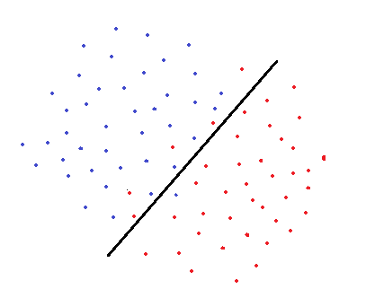import numpy as np
import pandas as pd

import matplotlib.pyplot as plt
%matplotlib inline

from sklearn.svm import SVC
from sklearn.datasets import make_classification

X, y = make_classification(n_samples=200, n_features=2,
n_informative=2, n_redundant=0, n_repeated=0, n_classes=2,random_state=42)

plt.figure(figsize=(10,6))
plt.title("Synthetic Binary Classification Dataset", fontsize=18)
plt.scatter(X[:,0], X[:,1], c=y, cmap='cool')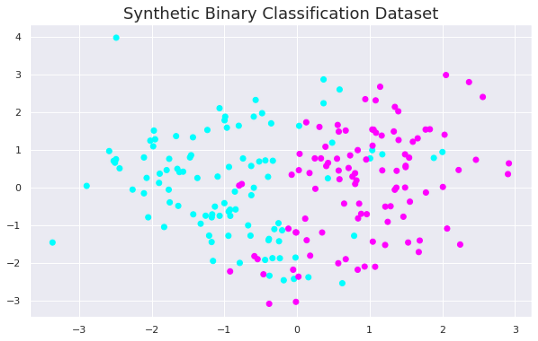clf = SVC(C=0.1, kernel='linear').fit(X, y)

plt.figure(figsize=(10,6))
plt.title("Linear kernel with C=0.1", fontsize=18)
plt.scatter(X[:, 0], X[:, 1], c=y, s=50, cmap='cool')
plot_svc_decision_function(clf)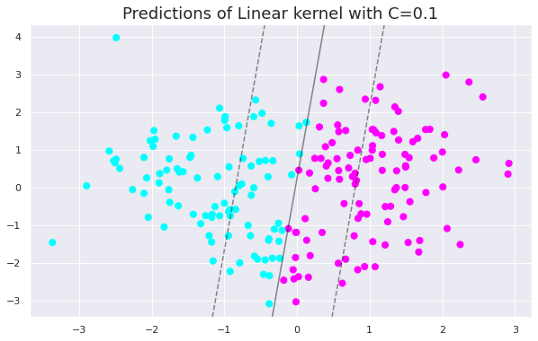Gamma是用于非线性支持向量机的超参数。最常用的非线性核函数之一是径向基函数（RBF）。RBF的Gamma参数控制单个训练点的影响距离。

gamma值较低表示相似半径较大，这会导致将更多的点组合在一起。对于gamma值较高的情况，点之间必须非常接近，才能将其视为同一组（或类）。因此，具有非常大gamma值的模型往往过拟合。

clf = SVC(C=1, kernel='rbf', gamma=0.01).fit(X, y)
y_pred = clf.predict(X)

plt.figure(figsize=(10,6))
plt.title("Predictions of RBF kernel with C=1 and Gamma=0.01", fontsize=18)
plt.scatter(X[:, 0], X[:, 1], c=y_pred, s=50, cmap='cool')
plot_svc_decision_function(clf)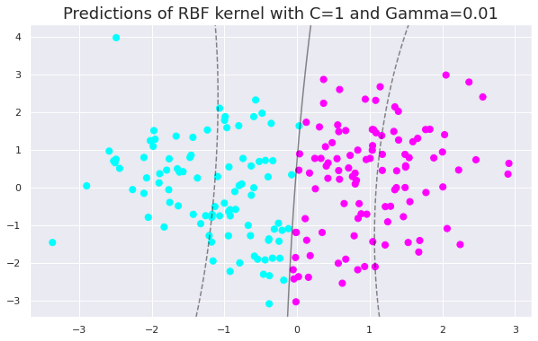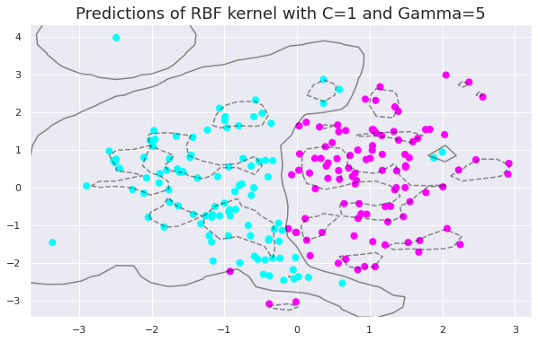#### gamma与C参数

0.0001 < gamma < 10

0.1 < c < 100

#### 参考引用

https://jakevdp.github.io/pythondastaciencemanual/05.07-support-vector-machines.html

http://panchuang.net/

sklearn机器学习中文官方文档：
http://sklearn123.com/

http://docs.panchuang.net/